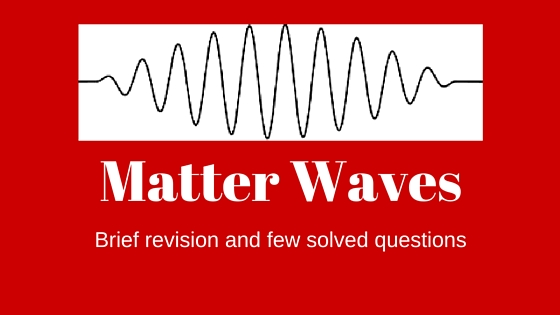# Matter Waves

This article is about matter waves and it contain brief introduction of the topic followed by few questions. Photoelectric effect and Compton scattering are evidences of the corpuscular nature of light. Interference , diffraction and polarisation reveal the wave nature of light. Louis de Brogli was the first one to suggest that matter may have wave like properties. He proposed that if light (which consists of waves classically) can sometimes behave as particles , then it should be possible for matter (which consists of particles classically) to exhibit wave-like behavioure under suitable circumstances.

According to de Brogli moving particle whatever its nature , has wave properties associated with it. Wavelength $\lambda$ associated with any particle of momentum $p$ is given by
$\lambda= \frac{h}{p} = \frac{h}{mv}$
From Plank’s theory of radiation , energy of photon is given by
$E = h\nu=\frac{hc}{\lambda}$
therefore     $\lambda =\frac{hc}{E}$
From special theory of relativity
$E=mc^{2}$
momentum of photon
$mc=p=\frac{h\nu}{c}=\frac{h}{\lambda}$
therefore     $p= \frac{h}{\lambda}$
or,$\lambda=\frac{h}{p}$
Thus what is true for energy packet (quanta or photon) is also true for material particle. Thus for a particle of mass $m$ moving with velocity $v$ , we have $p=mv$ and de Brogli wavelength associated with particle is
$\lambda = \frac{h}{mv}$
where $m = {{{m_0}} \over {\sqrt {1 – {{{v^2}} \over {{c^2}}}} }}$ is the relativistic mass of the particle.
Note that

1. If $m$ or $v$ is large , the de Brogli wavelength associated with the particle is small
2. The de Brogli wave associated with a material particle or photon is independent of any charge associated with it.
Thus we know that
$K = {1 \over 2}m{v^2}$
Therefore $mv = \sqrt {2mK}$
$\lambda = {h \over {\sqrt {2mK} }}$
If a charged particle carrying a charge $q$ is accelerated through a potential difference $V$ Volts , then kinetic energy
$K=qV$
Therefore de Brogli wavelength for a charged particle of charge $q$ and if it is accelerated through a potential difference of $V$ Volts is given by
$\lambda = {h \over {\sqrt {2mqV} }}$
3. When material particle like neutron is in thermal equilibrium at temperature $T$ , then most probable Kinetic energy is given by
$E=KT$
where $K=1.38 \times 10^{-23}$ $\frac{J}{K}$ is the Boltzman constant.
Therefore de Brogli wavelength of a material particle at temperature $T$ is
$\lambda = {h \over {\sqrt {2mKT} }}$

## Solved Numericals

QUestion 1 A ball of mass 10 gm has a velocity 1.0 m/s. Calculate the wavelength associated with it. Why is this wave nature is not observed in our daily observations. Given that $h=6.62 \times 10^{-32}$ $J-sec$

Solution de Brogli wavelength is given by
$\lambda = {m \over {mv}} = {{6.62 \times {{10}^{ – 32}}J/\sec } \over {10 \times {{10}^{ – 3}}Kgm \times 1m\sec }} = 6.62 \times {10^{ – 32}}m$
This wavelength is much smaller then the dimension of the ball. Therefore in such a case wave like properties of matter can not be observed in our daily observations.

Question 2
Find the de Brogli wavelength in Angstroms for an electron of energy $V$ eV
Solution
de Brogli wavelength is given by
$\lambda = {h \over {\sqrt {2mE} }} = {h \over {\sqrt {2mqV} }}$
given that $E=1.6 \times 10^{-19} V$ Joule
mass of electron is $9.1 \times 10^{-31}$ Kg
and plank’s constant is $h=6.62 \times 10^{-32}$ $J-sec$
putting these in above equation we have
$\lambda = {{12.28} \over {\sqrt V }}$ Angstroms

Question 3 Calculate the de Brogli wavelength associated with a proton moving with velocity equal to 1/20 of the velocity of light. [Answer: $1.5 \times 10^{7}$ m/sec]

Question 4 Find the energy of neutron in units of electron Volts whose de Brogli wavelength is $1$ Angstroms. Given the mass of neutron is $m=1.64 \times 10^{-34}$ J-sec. [Answer: $8.13 \times 10^{-2}$ eV]

Question 5 Show that the de Brogli wavelength for a particle of rest mass $m_{0}$ and charge $q$ , accelerated from rest through a potential difference of $V$ Volts relativistically is given by
$$\lambda = {h \over {\sqrt {\left[ {2{m_0}qV\left( {1 + {{qV} \over {2{m_0}{c^2}}}} \right)} \right]} }}$$
Solution
In this case Kinetic energy, $T=qV$
Relativistic formula for kinetic energy is
$$E = T + {m_0}{c^2} = qV + {m_0}{c^2}$$
So that ,
$${p^2}{c^2} = {E^2} – m_0^2{c^4} = {\left( {qV + {m_0}{c^2}} \right)^2} – m_0^2{c^4}$$
or,
$${p^2}{c^2} = {q^2}{V^2} + 2qV{m_0}{c^2}$$
$${p^2} = 2qV{m_0}\left[ {1 + {{qV} \over {2{m_0}{c^2}}}} \right]$$
or
$$p = \sqrt {2qV{m_0}\left[ {1 + {{qV} \over {2{m_0}{c^2}}}} \right]}$$
Therefor de Brogli wavelength is
$$\lambda = {h \over {\sqrt {\left[ {2{m_0}qV\left( {1 + {{qV} \over {2{m_0}{c^2}}}} \right)} \right]} }}$$

Subscribe
Notify ofThis site uses Akismet to reduce spam. Learn how your comment data is processed.